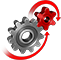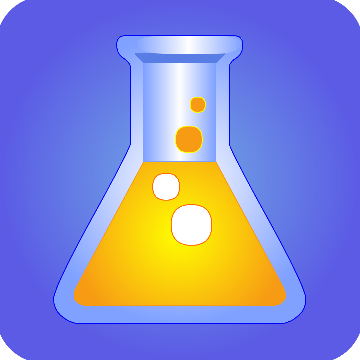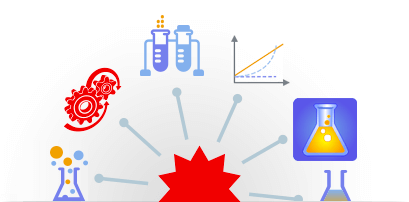# WolframSystemModeler

## System Modeler Modelica Library Store### High School Chemistry

Wolfram

The High School Chemistry library contains interactive chemistry labs for high-school students. After completing the labs, you will be able to calculate the final concentration of the products of any chemical reaction, calculate the solubility product of a heterogenous reaction and calculate the half-life time of a reaction. The library also contains many exercises related to rate laws, homogenous reactions and heterogenous reactions.

#### Features

• Dynamic simulation of chemical processes
• Exercises with real-life scenarios
• Compare scenarios by varying parameter valuesHigh School Chemistry Wolfram